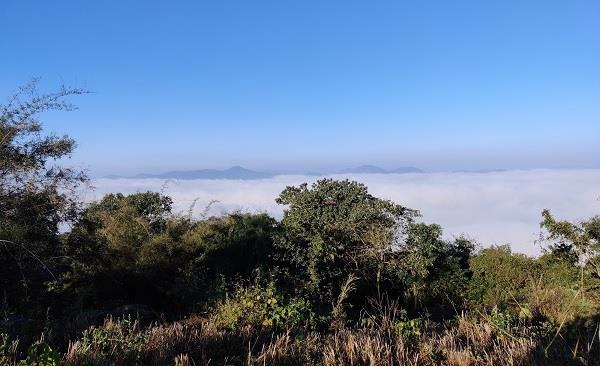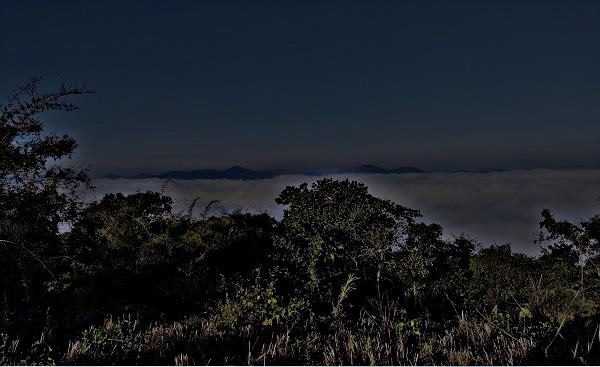# How to alter the sharpness of an image using Java OpenCV library?

Sharpening an image is the opposite of blur. To alter the sharpness of an image using the OpenCV library, you need to smooth/blur it using the Gaussian filter and subtract the smoothed version from the original image.

## Example

import org.opencv.core.Core;
import org.opencv.core.Mat;
import org.opencv.core.Size;
import org.opencv.imgcodecs.Imgcodecs;
import org.opencv.imgproc.Imgproc;
public class AlteringSharpness {
public static void main (String[] args) {
//Reading the Image from the file
String file ="D:\Image\lamma1.jpg";
//Creating an empty matrix
Mat dest = new Mat(src.rows(), src.cols(), src.type());
Imgproc.GaussianBlur(src, dest, new Size(0,0), 10);
Core.addWeighted(src, 1.5, dest, -0.5, 0, dest);
// Writing the image
Imgcodecs.imwrite("D:\Image\altering_sharpness_100.jpg", dest);
}
}

## Input## Output